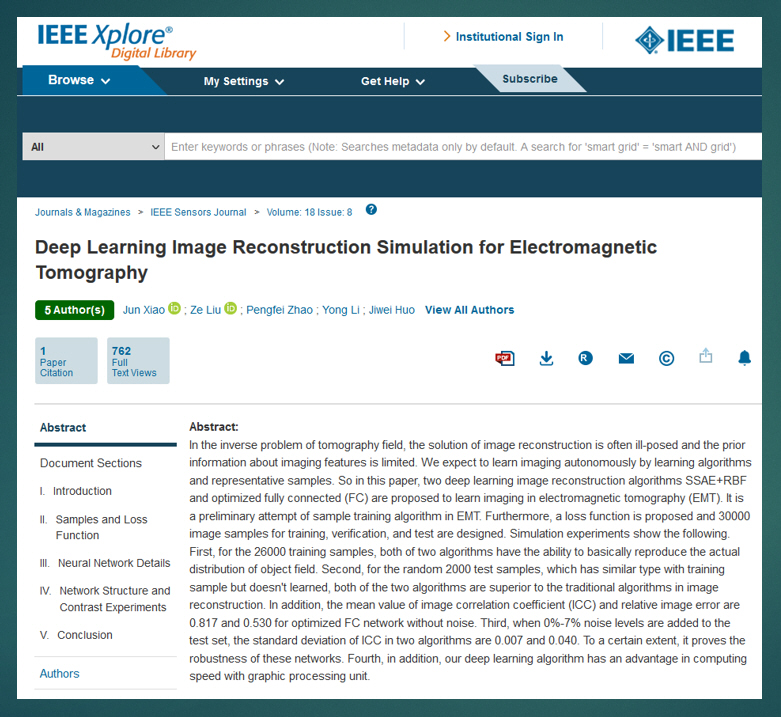# 科技论文-Deep Learning Image Reconstruction

2019-03-24 21:20:51

Deep Learning Image Reconstruction Simulation for Electromagnetic Tomography

http://dx.doi.org/10.1109/JSEN.2018.2809485Abstract:

In the inverse problem of tomography field, the solution of image reconstruction is often ill-posed and the prior information about imaging features is limited. We expect to learn imaging autonomously by learning algorithms and representative samples. So in this paper, two deep learning image reconstruction algorithms SSAE+RBF and optimized fully connected (FC) are proposed to learn imaging in electromagnetic tomography (EMT). It is a preliminary attempt of sample training algorithm in EMT. Furthermore, a loss function is proposed and 30000 image samples for training, verification, and test are designed. Simulation experiments show the following. First, for the 26000 training samples, both of two algorithms have the ability to basically reproduce the actual distribution of object field. Second, for the random 2000 test samples, which has similar type with training sample but doesn't learned, both of the two algorithms are superior to the traditional algorithms in image reconstruction. In addition, the mean value of image correlation coefficient (ICC) and relative image error are 0.817 and 0.530 for optimized FC network without noise. Third, when 0%-7% noise levels are added to the test set, the standard deviation of ICC in two algorithms are 0.007 and 0.040. To a certain extent, it proves the robustness of these networks. Fourth, in addition, our deep learning algorithm has an advantage in computing speed with graphic processing unit.